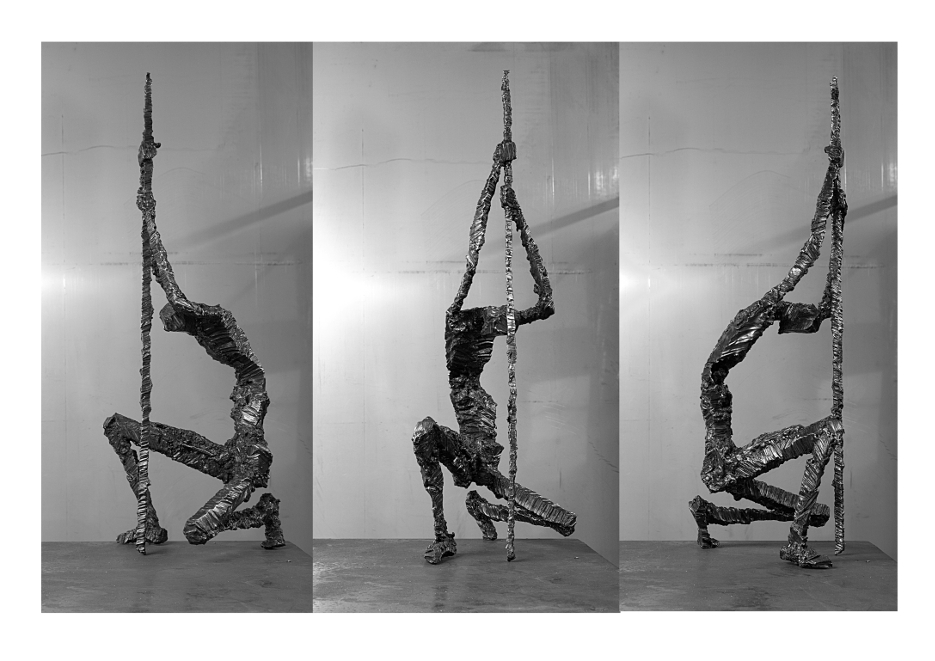F      L      A      M      E

C     U      T

M     I      L      D

S      T      E      E      L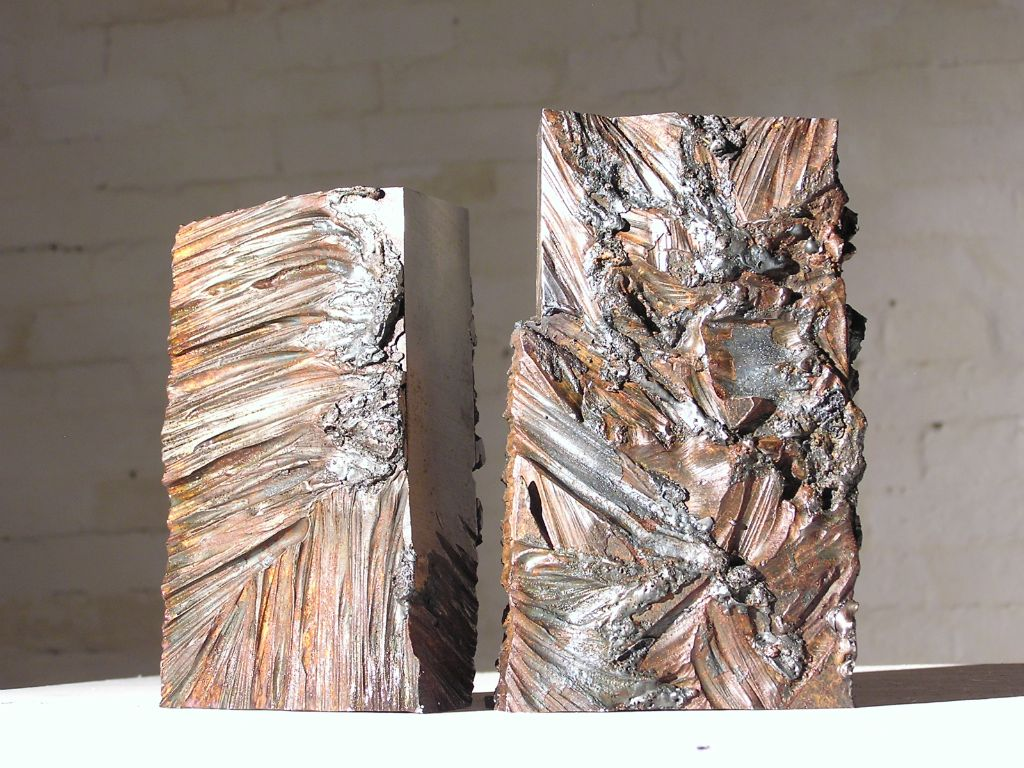Miniliths 2006

U S    W e s t  I n d i e s

U K     S P A I N

American born living in the UK

I use a welder's torch similar to someone working with clay uses a knife

work has evolved from functional to figurative to abstract over twenty years and now oscillates

smallish scale outweighs large as I tend not to make things I can't carry

P H O T O S

U K  c o l l e c t i o n  2 0 0 5 - p r e s e n t

c o m p l e t e  w o r k s  -  p u b l i c a t i o n

w e s t  i n d i e s  p o r t f o l i o  2 0 0 0 - 2 0 0 4

3  e a r l y  p i e c e s

p   h   o    t   o   s   t   r   e   a   mT E X T S / B L O G S

s p a i n - 2 0 1 0  g a r d e n  p r o j e c t

o t h e r  w o r k s

o u r o b o r o s   v i s e  -  E s s a y

p o l e p l a n t e r  -  E s s a y

m u s i n g s

p o e m s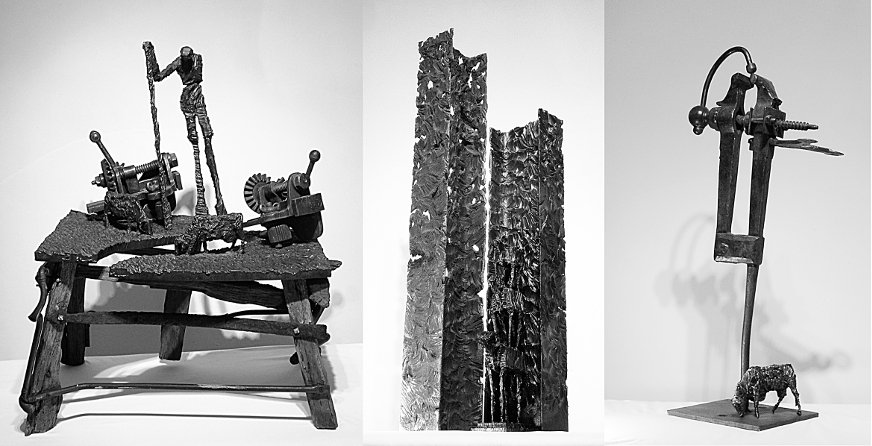A B O U T

CV/ E n v i r o n m e n t a l  p u r s u i t s

t y l e r f e n n @ g m a i l . c o m

a  v  a  i  l  a  b  l  e    o  n    S  I  N  G  U  L  A  R  T

'MAD COWS- The Cows Consume the Bucket that once Spit them to the Flames'

Twenty four hand carved cows with loader bucket, New York 2012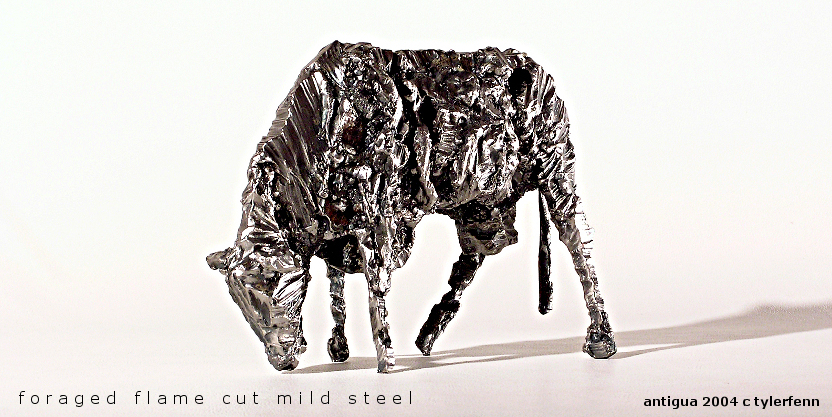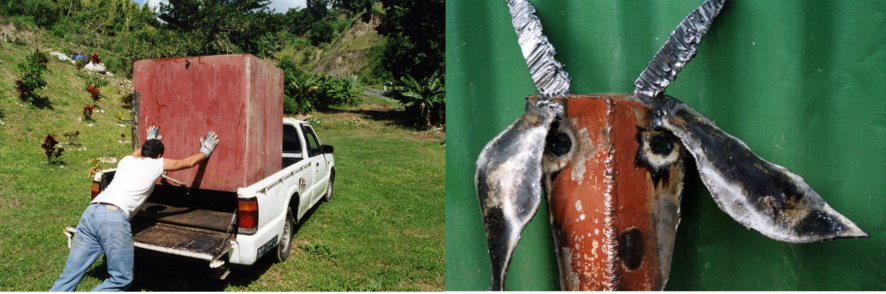UPCOMING SHOWING:        FLORENCE BIENNALE    OCTOBER   2021

Bitcoin:     35TqDkHCRn9o5NG4AwShYdXc5EwmE1Awso